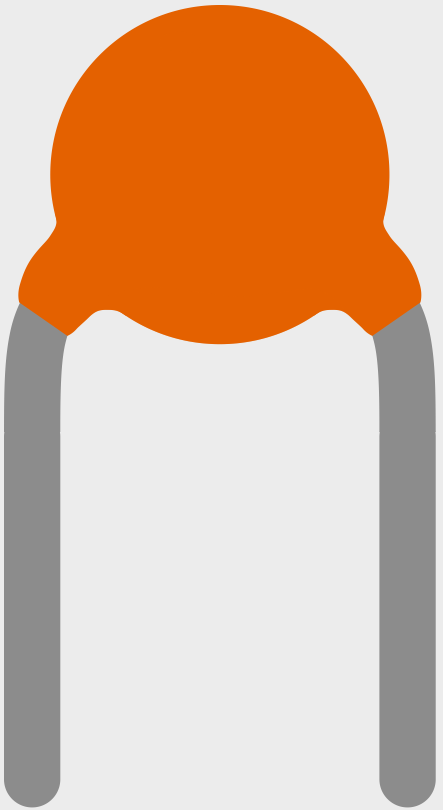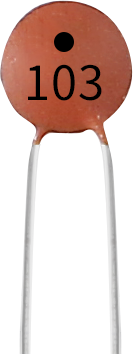Capacitor¶Capacitance, refers to the amount of charge storage under a given potential difference, denoted as C, and the international unit is farad (F). Generally speaking, electric charges move under force in an electric field. When there is a medium between conductors, the movement of electric charges is hindered and the electric charges accumulate on the conductors, resulting in accumulation of electric charges.

The amount of stored electric charges is called capacitance. Because capacitors are one of the most widely used electronic components in electronic equipment, they are widely used in direct current isolation, coupling, bypass, filtering, tuning loops, energy conversion, and control circuits. Capacitors are divided into electrolytic capacitors, solid capacitors, etc.

According to material characteristics, capacitors can be divided into: aluminum electrolytic capacitors, film capacitors, tantalum capacitors, ceramic capacitors, super capacitors, etc.

In this kit, ceramic capacitors are used. There are 103 or 104 label on the ceramic capacitors, which represent the capacitance value, 103=10x10^3pF, 104=10x10^4pF

Unit Conversion

1F=10^3mF=10^6uF=10^9nF=10^12pFExample